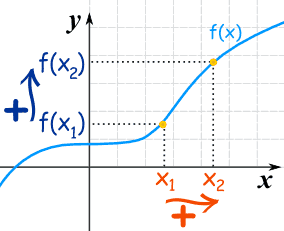×#### Thank you for registering.

One of our academic counsellors will contact you within 1 working day.

Click to Chat

1800-1023-196

+91-120-4616500

CART 0

• 0

MY CART (5)

Use Coupon: CART20 and get 20% off on all online Study Material

ITEM
DETAILS
MRP
DISCOUNT
FINAL PRICE
Total Price: Rs.

There are no items in this cart.
Continue Shopping

Dear Sir, For the function f(x)=X^x . Find the points at which it is 1.Increasing. 2. Decreasing . Hence Determine which of e^(pi) , (pi)^ e is greater .


9 years ago

							Dear student,
Increasing Functions
A function is "increasing" if the y-value increases as the x-value increases, like this:It is easy to see that y=f(x) tends to go up as it goes along.

What about that flat bit near the start? Is that OK?

Yes, it is OK if you say the function is Increasing
But it is not OK if you say the function is Strictly Increasing (no flatness allowed)

Using Algebra
What if you can't plot the graph to see if it is increasing? In that case is is good to have a definition using algebra.
For a function y=f(x):

when x1 < x2 then  f(x1) ≤ f(x2)
Increasing

when x1 < x2 then  f(x1) < f(x2)
Strictly Increasing

That has to be true for any x1, x2, not just some nice ones you choose.


9 years ago
							f(x) = xx

d/dx [f(x)] = d/dx [ xx ]

f1(x)       =xx [ 1 + logx ]
f1(x) = 0 , when 1+logx = 0 ,  xx cannot be 0..
1+ logx = 0
logx = -1
x = 1/e
if x > 1/e , then function is increasing
if x < 1/e , then function is decreasing
increasing => (1/e , infinity)
decreasing=> (-infinity,1/e)

9 years ago


9 years ago
Think You Can Provide A Better Answer ?

## Other Related Questions on Differential Calculus

View all Questions »### Course Features

• 731 Video Lectures
• Revision Notes
• Previous Year Papers
• Mind Map
• Study Planner
• NCERT Solutions
• Discussion Forum
• Test paper with Video Solution### Course Features

• 51 Video Lectures
• Revision Notes
• Test paper with Video Solution
• Mind Map
• Study Planner
• NCERT Solutions
• Discussion Forum
• Previous Year Exam Questions